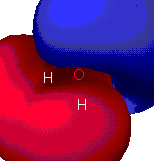# Theoretical Calculations of Properties of Water

by Dustyn Sawall

### Types of calculations performed

In general, Molecular orbitals (MO) are combinations of Atomic orbitals (AO). Atomic orbitals are actually mathematical expressions representing where a electron in a given quantum state can be found. When AO's are added together, they are done so in a way to minimize the energy of the system. This is done by some contributions of AO's being greater than others. The mathematical formula for this simplifies into three type of factors, the energy of the original AO, the energy saved by forming the bond and an overlap factor dependent on multiple interactions between electrons that need to be evaluated at the same time.

These equations rapidly become huge. To do them for all of the overlap considerations takes considerable time and computing power. Even then, they don't all work out. These are known as ab initio calculations. To improve workability of the mathematics, some modifications can be made. Generally, the more of the overlap integrals you use, the better your calc, but that is a trade off with if they can be done at all.

In the programs used for this page, three different levels of theory are analyzed. In the extended Huckel theory, it is assumed that only nearest neighbors interact. Zindo level of theory uses intermediate neglect of the overlap intergrals. It works using some empirical data from ab initio calculations to optimize its MO's. MOPAC level of theory uses a moderate neglect of these intergrals and uses empirical data from thermodynamics to improve it's theory. MOPAC is the most precise of the programs used because it takes into account more of the intergrals in question.

### Results of geometry optimization at various levels of theory

Initially, only a Molecular Mechanics program was used. This program treats the atoms as classical harmonic oscillator. The bond lengths and angles are calculated from rotational and vibrational spectroscopy results. The computer has tables of bond lengths, the computer then uses standard values from these tables to optimize the molecules. The molecule is designed so as to minimize the stress between atoms. This is the program used for the extended huckel level of theory. Zindo and MOPAC have geometry optimizations available to them from quantum theory to further optimize the molecules. In theory, the Mopac calc should be best. If the bond lengths and angles were examined in detail, there would be slight differences, but as can be seen from the pictures below, they are slight.

 Extended Huckel MOPAC ZINDO

### The molecular orbitals that provide the primary bonding

This is a picture of a MO that is providing bonding in this molecule. For water, our calculations turned up six different MO's, two of which were unfilled. This MO, consisting of the s orbitals from all three atoms is creating a sigma type bond across the entire molecule. The picture below was calculated using the Extended Huckel level of theory.### The highest occupied (HOMO) and lowest unoccupied (LUMO) molecular orbitals

These are the orbitals that are most involved in chemical reactions since they can most easily lose or gain electrons.Highest Occupied Molecular Orbital. This is the molecular orbital that is at the highest energy yet still has electrons in it. This orbital in particular is the z axis p orbital of the oxygen. It is a non-bonding orbital.Lowest Unoccupied Molecular Level This is a molecular orbital that does not have any electrons in it. As MO's are filled from lowest energy on up, there were no electrons to fill this high energy, anti-bonding orbital.

### Electron density/distribution mapsextended Huckel theoryMOPAC theoryZindo level of theory
These maps show the most probable locations for electrons in the molecule. The maps are read as you would a thermal imaging diagram. The hot colors(white, red, and yellow, ) are places where there is a high probability of finding an electron. The tendency of electrons to be in these locations give rise to a more negative charge in these areas.

### Charge distribution maps(Ext. HuckelMOPACZINDO
These maps show the relative distribution of positive and negative charge on the molecules. The red indicates a positive charge and blue is for a negative charge.

#### Partial atomic chargesoverhead veiwHydrogen end on veiw
As can be seen from these diagrams, there is a clear division of partial charges that will show a dipole characteristic. The red is for positive partial charge, blue for negative partial charge. Both of these pictures were calculated at the MOPAC level of theory.

### Calculated dipole moments

 chemical sample dipole moment (debye) water via Huckel calc. 2.647 water via Mopac calc. 1.739 water via Zindo calc. 2.211 water via CRC 1.854